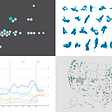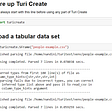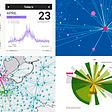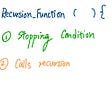# NumPy — Programming at Multi Dimensions.

`!pip install numpy`
`import numpy as npa = np.array([[1,2,3],[1,2,3],[4,5,6]])`
`array_zeros = np.zeros(4)`
`array_ones = np.ones(4)`
`array_empty = np.empty(4)`
`ip: i = np.identity(3)    iop:array([[1., 0., 0.],        [0., 1., 0.],        [0., 0., 1.]])`
`ip:array_a = np.array([[1,2],[3,4]])   array_b = np.array([[1,2],[3,4]])   dot_product = np.dot(array_a,array_b)   cross_product = np.cross(array_a, array_b)   dot_product   cross_productop: array([[ 7, 10],        [15, 22]])    array([0, 0])`
`ip: numpy_array = np.array([1, 2, 3, 4, 5, 6, 7, 8, 9])    mean = numpy_array.mean()    variance = numpy_array.var()    standard_deviation = numpy_array.std()    print('Mean :',mean)    print('Variance:',variance)    print('Standard Deviation:', standard_deviation)op: Mean : 5.0     Variance: 6.666666666666667     Standard Deviation: 2.581988897471611`

--

--

--

## More from Akshar Rastogi

Love podcasts or audiobooks? Learn on the go with our new app.

## “Where The Car Is Allergic To Vanilla Ice Cream And Exercise Causes Skin Cancer”## New Stunning Charts and Maps in Weekly Roundup — DataViz Weekly## How to find and organize your data from the command-line## Machine Learning Specialization with P## Simple Linear Regression In Python/NumPY## Social Distancing in Data Visualizations — DataViz Weekly## Is Airbnb a good side-hustle in 2022?## Engaging Data Graphics on Hobbies, Protests, Commute, Reddit — DataViz Weekly## Character Encoding basics: A.S.C.I.I.## SAP — Logging onto SAP System## Back to Basics with C